# Boats & Stream Tricks with QuestionsThe two most important and only elements in this type of problems is a BOAT and a STREAM. A stream is nothing but the moving water, which is sometimes also called CURRENT.
In the question, by speed of the boat (or swimmer), we mean the speed of the boat (or swimmer) in still water.

Upstream: When the boat (or swimmer) moves against i.e. in the opposite direction of the stream.

Downstream: when the boat (or swimmer) moves in the same direction as that of the stream.

Now, if the speed of the boat is B km/hr while the speed of the stream is S km/hr, then
Upstream speed U = (B – S) km/hr
Downstream speed D = (B + S) km/hr

If in the question, you are provided with the upstream and the downstream speed U and D respectively, to determine the speed of boat and stream the formula goes like
Speed of boat B = (D + U) / 2
Speed of stream S = (D - U) / 2
Also, always have with you the basic speed formula,Question: In still water, the speed of the boat is 7 km/hr. Find out the downstream speed of a boat in the river whose speed of the stream is 2 km/hr.
Solution: Downstream speed = Speed in still water + Speed of stream = 7 + 2 = 9 km/hr
A couple more formulae,

### Formula 1:

If the ratio of downstream and upstream speeds is D: U, then ratio of time taken will be U : D.

Question: A boat running upstream takes 8 hours 48 minutes to cover a certain distance, while it takes 4 hours to cover the same distance running downstream. What is the ratio between the speed of the boat and speed of the water current respectively?
Solution: When 8 hours 48 minutes is written using mixed fractions,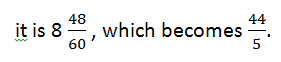Therefore, the ratio of the time taken becomesWhich on solving gives,Now, as the ratio of time is inversely proportional to that of Upstream and Downstream speeds.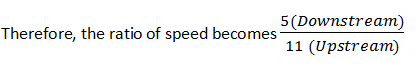Now, the ratio of speed of boat and the speed of stream will be### Formula 2:

When a boat’s speed in still water is B km/h and stream is flowing with a speed of S km/h and T is the time taken to row to a place and back, then the distance between the two placesQuestion: A boat’s speed in still water is 6 km/h while river is flowing with speed of 1.2 km/h and it takes 1 hour to row to a place and back. How far is the place?
Solution: Take out the formula book

### Formula 3:

A man rows a certain distance downstream in X hrs and returns the same distance in Y hrs. If the stream flows at the rate of Z km/hr then the speed of the boat in still water is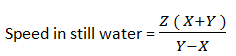Question: Ramesh can row a certain distance downstream in 6 hrs and returns the same distance in 9 hrs. If the stream flows at the rate of 3 km/hr, find the speed of Ramesh in still water.
Solution: Consulting the formula book,

### Formula 4:

A man rows a certain distance downstream in X hrs and returns the same distance in Y hrs. If the speed of the man in still water is Z km/hr then the speed of the stream will be1. In still water speed of boat is 8 km/hr. Find the upstream speed of boat which is floating in the sea where speed of waves is 3 km/hr.

Solution: Speed of the boat (B) = 8
Speed of the stream (S) = 3
Upstream speed = B – S = 8 – 3 = 5 km/hr (answer)

2. A boat covers a distance of 30 km in 3 hrs in the direction of stream and covers same distance in 5 hrs in opposite direction of the stream. Find speed of the boat in still water.3. In still water, speed of boat is 10 km/hr. Speed of the stream is 4 km/hr. Therefore, in how much time will it cover a distance of 70 km downstream?

Solution: Speed of the boat (B) = 10
Speed of the stream (S) = 4
Therefore, Downstream speed = B + S = 10 + 4 = 14 km/hr
Now, Speed (Downstream) = 14
Distance (Downstream) = 70
And according to what the formula says,X = = 5 hrs (answer)

4. The speed of a boat in downstream is 24km/hr and speed of the stream is 8 km/hr. find the speed of the boat in still water.

Solution: Downstream speed = 24
Speed of the stream (S) = 8
According to the formula,
Downstream = B + S
24 = B + 8
Speed of the Boat (B) = 24 – 8 = 16 km/hr (answer)

5. The speed of a boat is 18 km/hr in upstream and the speed of the stream is 6 km/hr. find out the speed of the boat in downstream.

Solution: Upstream speed = 18
Speed of the stream (S) = 6
According to the formula,
Upstream = B - S
18 = B – 6
Speed of the boat (B) = 24
Now,
Downstream = B + S = 24 + 6 = 30 km/hr (answer)

6. The speed of a man is 18 km/hr downstream and 10 km/hr upstream. Find out his speed in still water.7. A man can row with 15 km/hr in still water and the speed of the stream is 13 km/hr. How much distance does he cover in 2 hrs during upstream?8. The speed of a ship is 50 km/hr in downstream and 30 km/hr in upstream. How much time will it take to cover 280 km in still water?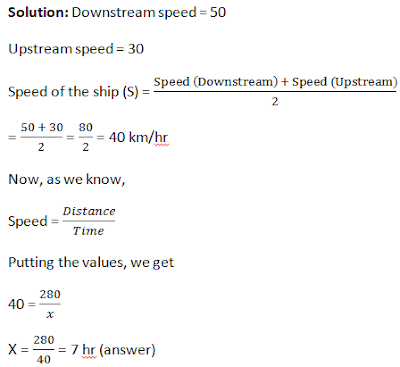9. The speed of a man in still water is 6 km/hr and the speed of the stream is 1.5 km/hr. A man rows to a place at a distance of 22.5 km and comes back to the starting point. Find the total time taken by him.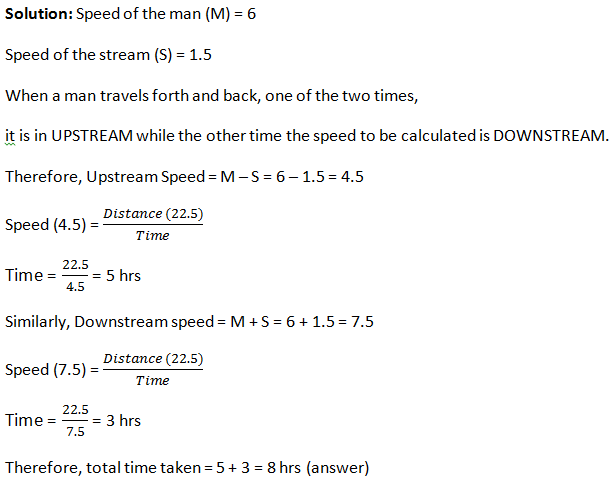10. A man rows upstream 16 km and downstream 28 km taking 5 hours each time. Find the velocity of the current.

### Practice Questions

1. A man rows upstream 16 km and downstream 28 km taking 5 hours each time. Find the velocity of the current.
2. A motorboat takes 4 hours to travel a distance of 22 km downstream and it takes 8 hours to travel the same distance upstream. Find the difference between the speed of the boat in still water and that of the current.
3. A boat takes 3 hours to cover a certain distance when going with the stream and 5 hrs to return to the starting point. The rate of the flow of river is 5 km/hr. Find out the speed of the boat in still water.
4. A boat can go 15.2 km downstream in 24 minutes. If the speed of the stream is 2.5 km/hr. How much distance (in km) can the boat cover upstream in 18 minutes?
5. A boat can travel 4.2 km upstream in 14 minutes. If the ratio of the speed of the boat in still water to the speed of the stream is 7 : 1. How much time (in minutes) will the boat take to cover 17.6 km downstream?
6. If a man’s rate with the current is 12 km/hr and rate of the current is 1.5 km/hr. Find the rate of the man against the current?
7. A boat travels upstream from B to A and downstream from A to B in 3 hrs. If the speed of the boat in still water is 9 km/hr and the speed of the current is 3 km/hr, find the distance between A and B.
8. A can row a certain distance downstream in 6 hrs and return the same distance in 9 hrs. If the stream flows at the rate ofkm/hr, find how far he can row in an hour in still water.
9. A man can row 40 km upstream and 55 km downstream in 13 hrs. Also, he can row 30 km upstream and 44 km downstream in 10 hrs. Find the speed of the man in still water and the speed of the current.
10. A man can row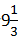km/hr in still water and he finds out that it takes him thrice as much time to row up than as to row down the same direction in river. The speed of the current is?

These are the type of questions usually seen in the examinations. Try solving them using the formulas above and become a pro in no time. Let us know below in the comment section if you find difficulty in solving any of the questions here.

**BEST OF LUCK **#### What's trending in BankExamsToday

Smart Prep Kit for Banking Exams by Ramandeep Singh - Download here